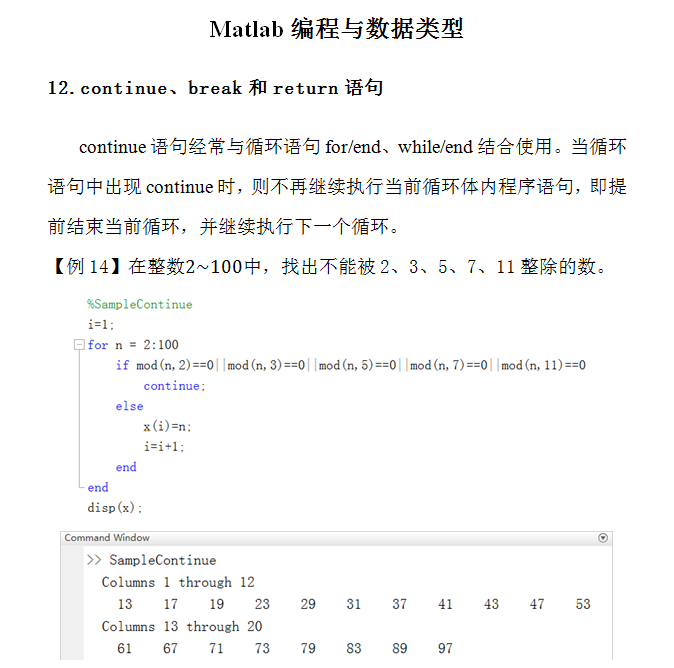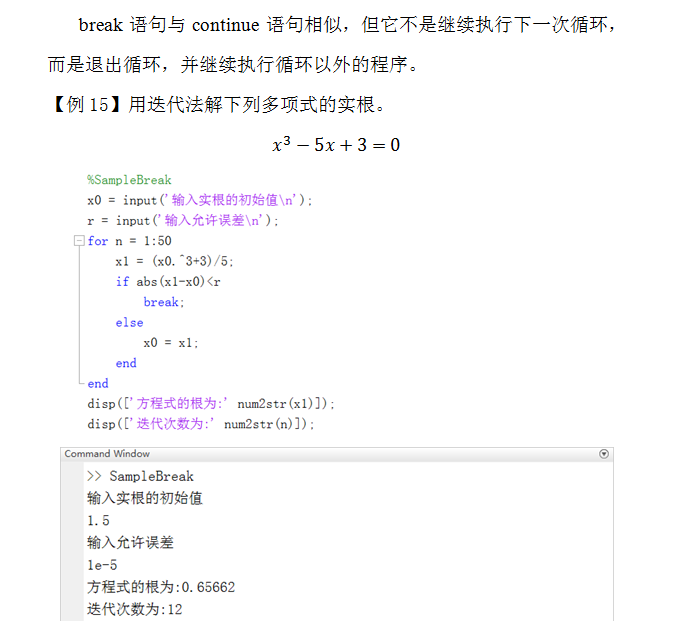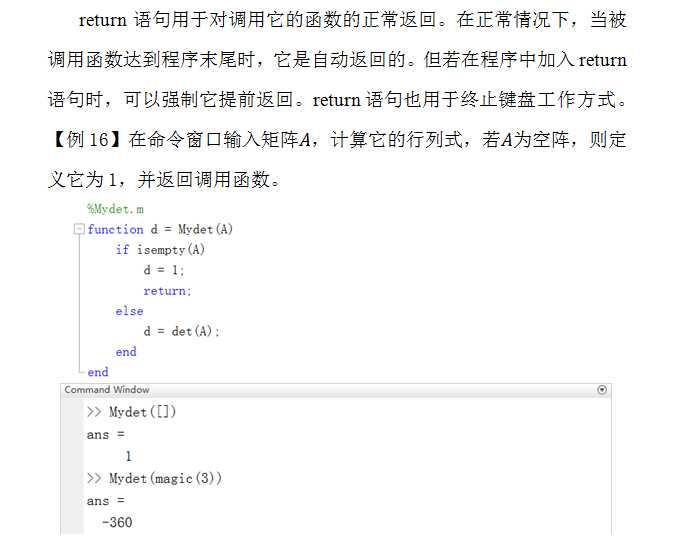• ## Matlab中的continue、break和return语句

千次阅读 多人点赞 2020-01-06 16:54:01
经常用在for和while语句中，continue一旦被执行，就会终止当前循环，进行下一次循环。 eg, 在for 循环： clc clear all close all i = 1; COND = 1; for i = 1:20 if mod(i,2)==0 continue else fprintf('...
Continue:
经常用在for和while语句中，continue一旦被执行，就会终止当前循环，进行下一次循环。
eg, 在for 循环中：
clc
clear all
close all

i = 1;
COND  = 1;

for i = 1:20
if mod(i,2)==0
continue
else

fprintf('the array is %d\n',i)
end
end

结果是：
the array is 1
the array is 3
the array is 5
the array is 7
the array is 9
the array is 11
the array is 13
the array is 15
the array is 17
the array is 19

我们跳过了能够被2整除的数字，找出来了剩下的数字。
eg, 在while 循环中：
clc
clear all
close all

i = 1;
COND  = 1;

while COND ==1

if mod(i,2)==0
i = i+1;
continue;
else
fprintf('the array is %d\n',i)
end

if i>=19
COND = 2;
end
i = i+1;
end

这里可以看到，我们在符合while条件下的循环中，如果被2整除了，那么就会跳过这个循环，直接进到下一个while循环下，从头开始执行。再一次进入if 判断新的i是否满足整除要求。
结果如下：
the array is 1
the array is 3
the array is 5
the array is 7
the array is 9
the array is 11
the array is 13
the array is 15
the array is 17
the array is 19

break:
break和continue用法相似，区别在于，一旦执行了break, 就会推出循环，而不是进入下一个循环：
在for循环中：
我们在1:10中，到一个被3整除的，一旦找到了第一个，整个for循环就不会再执行了。但是要注意，只是不执行break所在的循环，其他的循环还是要执行的。
clc
clear all
close all

i = 1;
COND  = 1;

for i = 1:10
if mod(i,3)==0
break
else

fprintf('the array is %d\n',i)
end
end

结果为：
the array is 1
the array is 2

如果我们人工的在添加一个for 循环：
%%
clc
clear all
close all

i = 1;
COND  = 1;

for j = 1:4

for i = 1:j
if mod(i,3)==0
break
else

fprintf('the array is %d\n',i)
end
end
end

结果为：
the array is 1
the array is 1
the array is 2
the array is 1
the array is 2
the array is 1
the array is 2

我们会把外层的for 循环全部执行完。
return:
return 跳出函数，返回调用函数处。
无论是while还是for,直接跳出函数，返回到调用函数之前。
%%
clc
clear all
close all

i = 1;
COND  = 1;

for i = 1:20
if mod(i,2)==0
return
else

fprintf('the array is %d\n',i)
end
end
%%
clc
clear all
close all

i = 1;
COND  = 1;

while COND ==1

if mod(i,2)==0
i = i+1;
return;
else
fprintf('the array is %d\n',i)
end

if i>=19
COND = 2;
end
i = i+1;
end

执行这两段函数都是一个结果：
the array is 1
>>

当i = 2时，函数就跳出了。
用一个更简单的例子说明，我们有一个很多层的if else 判断语句，这个时候用return 就很好，如果符合条件就可以直接跳出多层if else语句，而不需要把所有的if else 全部执行一遍再跳出。
clc
clear all
close all
flag = 20 ;
if flag <-10
fprintf('y<-10\n')
return
elseif flag >20
fprintf('y>20\n')
return
elseif flag >=-10 && flag <20
fprintf('-10<=y<20\n')
return
else fprintf('y=20\n')
return
end

总结来说：
break       跳出循环；
continue    继续循环，（不执行 循环体内continue 后面的语句，直接进行下一循环）
return      跳出函数，返回调用函数 处。

刚才的代码放在一起方便复制：
clc
clear all
close all

i = 1;
COND  = 1;

for i = 1:20
if mod(i,2)==0
continue
else

fprintf('the array is %d\n',i)
end
end
%%
clc
clear all
close all

i = 1;
COND  = 1;

while COND ==1

if mod(i,2)==0
i = i+1;
continue;
else
fprintf('the array is %d\n',i)
end

if i>=19
COND = 2;
end
i = i+1;
end
%%
clc
clear all
close all

i = 1;
COND  = 1;

for i = 1:4
if mod(i,3)==0
break
else

fprintf('the array is %d\n',i)
end
end

%%
clc
clear all
close all

i = 1;
COND  = 1;

for j = 1:4

for i = 1:j
if mod(i,3)==0
break
else

fprintf('the array is %d\n',i)
end
end
end
%%
clc
clear all
close all

i = 1;
COND  = 1;

for i = 1:20
if mod(i,2)==0
return
else

fprintf('the array is %d\n',i)
end
end
%%
clc
clear all
close all

i = 1;
COND  = 1;

while COND ==1

if mod(i,2)==0
i = i+1;
return;
else
fprintf('the array is %d\n',i)
end

if i>=19
COND = 2;
end
i = i+1;
end
%%
clc
clear all
close all
flag = 20  ;
if flag <-10
fprintf('y<-10\n')
return
elseif flag >20
fprintf('y>20\n')
return
elseif flag >=-10 && flag <20
fprintf('-10<=y<20\n')
return
else fprintf('y=20\n')
return
end




展开全文在一般程序语言中，return和break有特定的使用区域，不过在Matlab中return和break可以写成主语句，并不一定费得写在函数中或循环语句中。这一点和其它语言有很大的不同。

A=imread('Image15.jpg');
C=B-A;
imwrite(C,'compare.jpg');
figure,imshow(A);
break;
figure,imshow(B);
figure,imshow(C);
展开全文• 本微信图文详细介绍了Matlab中的continue、break和return语句
本微信图文详细介绍了Matlab中的continue、break和return语句。展开全文continue break
• 在编写MATLAB程序过程中，有时会遇到当程序运行到不满足if条件时让程序跳出，停止运行情况，在MATLAB中，使用return语句实现程序跳出。 a = 1; % 定义一个变量a flag = 1; % 定义一个标准量 if 1 if flag == a...
        在编写MATLAB程序过程中，有时会遇到当程序运行到不满足if条件时让程序跳出，停止运行的情况，在MATLAB中，使用return语句实现程序跳出。

a = 1;                  % 定义一个变量a
flag = 1;              % 定义一个标准量
if 1
if flag == a       %判断a与flag是否相等
disp('0');    %如果相等，命令行打印0
return;       %不再向下执行
disp('1');    %return 后的语句不执行
else
disp('2');    %如果不相等，命令行打印2
end
else
disp('3');        %外层if对应的else，命令行打印3，
end
disp('4');            %命令行打印4

程序运行后，命令行窗口打印0，可以证明在程序执行return后，以后的程序都不运行。

只将以上程序中变量a的值变为0，程序运行后，命令行窗口打印2,4。

只将以上程序中第一个if后的1变为0，程序运行后，命令行窗口打印3,4。

以上程序则可以清楚的验证，执行了return语句后，程序不再运行下去。


展开全文• 1.有选择地显示循环中的值 2.跳至下一循环迭代 将控制权传递给for或while循环的下一迭代。 一.语法 continue 提示 continue语句跳过for或while循环中剩余的说明，并开始下一迭代。要完全退出循环，请...
• 一 自定义函数时,用return语句指定返回值,即函数运行最后得出结果,举个例子:def Add(x,y): z = x+y return zdef Ad(x,y): z = x+y return x上边是我定义两个函数,除了名字不一样之外,唯一不同就是return后...
• return跳出整个函数，函数中return之后代码都不执行； break跳出最近循环语句； continue跳出循环当前执行，继续从头执行下一次循环
• 在编写MATLAB程序过程中，有时会遇到当程序运行到满足if条件时让程序跳出，停止运行情况，在MATLAB中，使用return语句或者空语句实现程序跳出。如果使用return语句，在脚本文件会出现小问题，在函数文件中则会正常...
• 经常会碰到提前终止循环，跳出子程序、显示出错信息等情况，这时就要用到一些用于控制程序流语句，在MATLAB中提供控制语句有continue、break、try-catch、return语句。1) continue 命令和break语语言
• 1、fgetl tline=fgetl(fid) 从文件中读取行，删除文件换行符(换行符和回车符) 返回由文件标识符fid指示文件下一行。...matlab中fgetl、fgets、fread函数 2、return语句 return 语句终止当前命令序列，...
• return语句表示结束函数执行。 调用格式： [输出实参表]=函数名（输入实参表） 在调用函数时，函数输入输出参数称为实际参数，简称实参。 匿名函数基本格式 函数句柄变量=@（匿名函数输入参数） 匿名函数表达式 ...
• 当需要重新加载修改后自定义 Python 模块时，需要在 MATLAB 工作空间删除所有变量，脚本和类。具体过程如下 创建 Python 模块 用 MATLAB 编辑器在当前文件夹新建文件 复制下述语句定义 myfunc 函数，...Python 混合编程
• 说明 Application of ... 3 关于 return 语句 如果在函数文件插入 了 return 语句则执行到该语句就结束函数 执行流程转至调用该函数位置通 常也不使用 return 语句 例 5.10 编写函数 文件求半径为 r 面积
• ## matlab if

千次阅读 2019-08-04 21:08:24
在编写MATLAB程序过程中，有时会遇到当程序运行到不满足if条件时让程序跳出，停止运行情况，在MATLAB中，使用return语句实现程序跳出。 只将以上程序中变量a值变为0，程序运行后，命令行窗口打印2,4。 只将...
• * Application of Matlab Language * matlab基础知识精心整理 与在线帮助文本相隔一空行注释行 包括函数文件编写和修改信息如作者和版本等 3关于return语句 如果在函数文件插入了return语句则执行到该语句就...
• MATLAB中的程序流程控制语句有： 1.分支控制语句（if结构和switch结构） 2.循环控制语句（for循环、while循环、continue语句和break语句） 3.程序终止语句（return语句） 分支控制语句： 分支语句可以使用程序中的一...
• ## Java跳转控制语句

千次阅读 2012-01-06 09:21:59
Java中没有goto语句，却有可以实现跳转功能的语句。分别是break语句，continue语句，return语句，和try catch finally语句。其中finally语句无论遇到哪种跳转，总终都会执行。...注： goto语句就像Matlab中的gljava 编译器 算法 语言# matlab中的return语句matlab 订阅